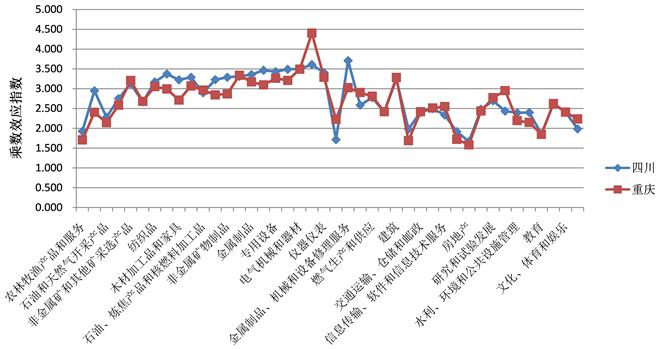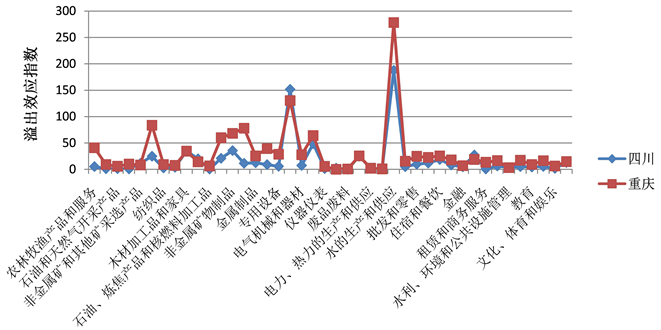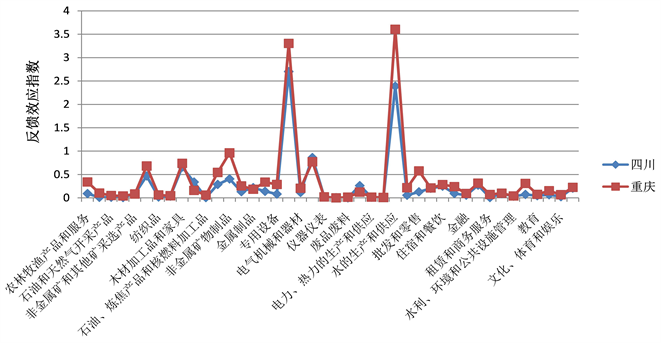#### 期刊菜单

Research on Industrial Spillover and Feedback Effect in Chengdu-Chongqing Region Based on Input-Output Model

Abstract: The two-regional input-output model is used to compile the inter-regional input-output table of Chengdu and Chongqing, and the input-output analysis method is used to measure, analyze and compare the intra-regional multiplier effect, interregional spillover effect and interregional feed-back effect of economic development in Chengdu and Chongqing. The research shows that the intensity of intra-regional industrial linkages in Sichuan Province is stronger, and the intensity of inter-regional industrial linkages in Chongqing is stronger. Chengdu-Chongqing region has industrial homogeneous competition and market segmentation in economic development. Therefore, the two places should build a high-end core industrial chain, promote the integrated development of the market, and strengthen policy coordination and cooperation.

1. 引言

2020年10月16日召开的中共中央政治局提出：“成渝地区双城经济圈的建设有利于形成优势互补、高质量发展的区域经济布局，是构建以国内大循环为主体、国内国际双循环相互促进的新发展格局的一项重大举措”。2021年10月20日，《成渝地区双城经济圈建设规划纲要》发布，并成为了成渝双城经济圈建设的纲领性文件以及制定相关规划和政策的重要依据，势必要将其打造为国家第四个重要经济圈，在西部形成高质量发展的重要增长极，如今关于两地经济产业的联系和联合发展越来越深入，由此两地的产业关联以及相关效应的研究也越来越得到重视。本文运用两区域投入产出模型对成渝两地产业关联进行分析的同时，也将其具体分解为地区间产业溢出效应和反馈效应进行分析和测算，提出相关的政策建议的同时以求进一步推动成渝地区双城经济圈的建设发展。

2. 两区域投入产出模型

$\left[\begin{array}{cc}{A}^{11}& {A}^{12}\\ {A}^{21}& {A}^{22}\end{array}\right]\left[\begin{array}{c}{X}^{1}\\ {X}^{2}\end{array}\right]+\left[\begin{array}{c}{Y}^{1}\\ {Y}^{2}\end{array}\right]=\left[\begin{array}{c}{X}^{1}\\ {X}^{2}\end{array}\right]$ (1)

Round (1985)  提出里昂惕夫逆矩阵并进整理和更改，简写为：

${X}^{1}={F}^{11}{L}^{11}{Y}^{1}+{F}^{11}{S}^{12}{L}^{22}{Y}^{2}$ (2)

${L}^{11}={\left(I-{A}^{11}\right)}^{-1}$ (3)

${L}^{22}={\left(I-{A}^{22}\right)}^{-1}$ (4)

${S}^{12}={\left(I-{A}^{11}\right)}^{-1}{A}^{12}$ (5)

${F}^{11}={\left[I-{\left(I-{A}^{11}\right)}^{-1}{A}^{12}{\left(I-{A}^{22}\right)}^{-1}{A}^{21}\right]}^{-1}={\left(I-{S}^{12}{S}^{21}\right)}^{-1}$ (6)

(3)式中的 ${L}^{11}$ 为区域1的里昂惕夫逆矩阵，表示区域1的乘数效应；(5)式中的 ${S}^{12}$ 表示为区域2对区域1的溢出效应；(6)式中的 ${F}^{11}$ 表示为区域1的反馈效应。两区域间各自的溢出效应相乘得出的 ${S}^{12}{S}^{21}$ 代表总产出变化的相互影响。由此得出的 ${\left(I-{S}^{12}{S}^{21}\right)}^{-1}$ 表示包含直接影响和间接影响的全部反馈效应。

$\left[\begin{array}{c}{X}^{1}\\ {X}^{2}\end{array}\right]=\left[\begin{array}{cc}{F}^{11}& 0\\ 0& {F}^{22}\end{array}\right]\left[\begin{array}{cc}I& {S}^{12}\\ {S}^{21}& I\end{array}\right]\left[\begin{array}{cc}{L}^{11}& 0\\ 0& {L}^{22}\end{array}\right]\left[\begin{array}{c}{Y}^{1}\\ {Y}^{2}\end{array}\right]$ (7)

$\begin{array}{c}\left[\begin{array}{c}{X}^{1}\\ {X}^{2}\end{array}\right]=\left\{\left[\begin{array}{cc}{L}^{11}& 0\\ 0& {L}^{22}\end{array}\right]+\left[\begin{array}{cc}0& {S}^{12}\\ {S}^{21}& 0\end{array}\right]\left[\begin{array}{cc}{L}^{11}& 0\\ 0& {L}^{22}\end{array}\right]+\left[\begin{array}{cc}{F}^{11}-I& 0\\ 0& {F}^{22}-I\end{array}\right]\left[\begin{array}{cc}I& {S}^{12}\\ {S}^{21}& I\end{array}\right]\left[\begin{array}{cc}{L}^{11}& 0\\ 0& {L}^{22}\end{array}\right]\right\}\left[\begin{array}{c}{Y}^{1}\\ {Y}^{2}\end{array}\right]\\ =\left[\begin{array}{cc}{L}^{11}{Y}^{1}& 0\\ 0& {L}^{22}{Y}^{2}\end{array}\right]+\left[\begin{array}{c}\left({F}^{11}-I\right){L}^{11}{Y}^{1}+\left({F}^{11}-I\right){S}^{12}{L}^{11}{Y}^{1}\\ \left({F}^{22}-I\right){S}^{12}{L}^{11}{Y}^{1}+\left({F}^{22}-I\right){L}^{22}{Y}^{2}\end{array}\right]\end{array}$ (8)

(2007)  将其进一步改进得出：

${L}^{rr}={\left(I-{A}^{rr}\right)}^{-1}$ (9)

${S}^{rr}{L}^{rr}={\left(I-{A}^{rr}\right)}^{-1}{A}^{rr}{L}^{rr}$ (10)

$\left({F}^{rr}-I\right){L}^{rr}=\left[{\left(I-{S}^{12}{S}^{21}\right)}^{-1}-I\right]{L}^{rr}$ (11)

$S{O}^{tr}=e{S}^{tr}{L}^{rr}$ (12)

$F{B}^{rr}=e\left({F}^{rr}-I\right){L}^{rr}$ (13)

3. 数据来源与区域间投入产出表编制说明

3.1. 数据来源

3.2. 区域间投入产出表编制说明

3.2.1. 区域间流量的估算和调整

${t}_{i}^{RS}=\frac{{X}_{i}^{R}{d}_{i}^{s}}{{\sum }_{R}{X}_{i}^{R}}{Q}^{RS}$ (14)

${Q}^{RS}=\frac{{H}^{RS}}{\frac{{H}^{RO}{H}^{OS}}{{H}^{OO}}}$ (15)

3.2.2. 系数矩阵的计算

$CAX+CF+E-M=X$ (16)

${a}_{ij}={X}_{ij}/{X}_{j}$ (17)

${C}_{i}^{RS}={t}_{i}^{RS}/{\sum }_{R}{t}_{i}^{RS}$ (18)

3.2.3. 区域间投入产出表的编制

4. 实证分析

4.1. 成渝乘数、溢出和反馈效应汇总分析Table 1. Summary of Chengdu-Chongqing multiplier effect, spillover effect and feedback effect

4.2. 成渝间各产业乘数、溢出和反馈效应比较研究

4.2.1. 区域内乘数效应Table 2. Measurement of multiplier effect in 42 industrial regions of Sichuan province and Chongqing cityFigure 1. Multiplier effect of 42 industrial sectors in Sichuan and Chongqing

4.2.2. 区域间溢出效应Table 3. Measurement of inter-regional spillover effects of 42 industries in Sichuan and ChongqingFigure 2. Spillover effects of 42 industrial sectors in Sichuan and Chongqing

4.2.3. 区域间反馈效应Table 4. Linkage measure of inter-regional feedback effect of 42 industries in Sichuan province and Chongqing cityFigure 3. Feedback effect of 42 industrial sectors in Sichuan and Chongqing

5. 结论和政策建议

  Miller, R.E. (1963) Comments on the “General Equilibrium” Model of Professor Moses. Macroeconomic, 15, 82-88. https://doi.org/10.1111/j.1467-999X.1963.tb00301.x  Round, J.I. (1985) Decomposing Multipliers for Eco-nomics Systems Involving Regional and World Trade. Economics Journal, 95, 383-399. https://doi.org/10.2307/2233216  潘文卿, 李子奈. 中国沿海与内陆间经济影响的反馈与溢出效应[J]. 经济研究, 2007, 42(5): 68-77.  吴福象, 朱蕾. 中国三大地带间的产业关联及其溢出和反馈效应——基于多区域投入-产出分析技术的实证研究[J]. 南开经济研究, 2010(5): 140-152.  黄庆华, 胡梦佳. 成渝地区双城经济圈产业关联演化格局分析[J]. 中南大学学报(社会科学版), 2021, 27(6): 119-135.  崔建刚, 孙宁华. 产业关联、结对扶贫与区域协调发展——对江浙沪及其帮扶地区的投入-产出分析[J]. 经济问题, 2019(3): 87-94+103.  孙凌宇, 罗杨帆. 川渝地区经济增长的空间溢出效应——基于区域间投入产出表的分析[J]. 重庆理工大学学报(社会科学), 2021, 35(8): 37-48.  侯璐璐, 张燕华. 地区经济主导产业与优势产业选择比较研究——以重庆市制造业为例[J]. 技术经济与管理研究, 2015(12): 113-117.  刘李佳, 王浩宇. 基于投入产出模型的京津冀区域产业溢出及反馈效应研究[J]. 经济问题, 2018(7): 123-128, 封3.